Computational & Technology Resources
an online resource for computational,
engineering & technology publications
Civil-Comp Proceedings
ISSN 1759-3433
CCP: 84
PROCEEDINGS OF THE FIFTH INTERNATIONAL CONFERENCE ON ENGINEERING COMPUTATIONAL TECHNOLOGY
Edited by: B.H.V. Topping, G. Montero and R. Montenegro
Paper 183

Stochastic Stability of Linear and Nonlinear Fluid Structure Coupled System with Non-Gaussian Multiplicative Noise

F. Poirion1, B. Puig2 and I. Zentner3

1French Aeronautics and Space Research Center, Chatillon, France
2Mathematical Laboratory, University of Pau, France
3France Electricity, EDF, Clamart, France

Full Bibliographic Reference for this paper
F. Poirion, B. Puig, I. Zentner, "Stochastic Stability of Linear and Nonlinear Fluid Structure Coupled System with Non-Gaussian Multiplicative Noise", in B.H.V. Topping, G. Montero, R. Montenegro, (Editors), "Proceedings of the Fifth International Conference on Engineering Computational Technology", Civil-Comp Press, Stirlingshire, UK, Paper 183, 2006. doi:10.4203/ccp.84.183
Keywords: aeroelasticity, non-Gaussian, random dynamical system, stability.

Summary
In a previous paper  the stability of fluid-structure coupled systems in turbulent flow has been investigated under the assumption that turbulence was introduced as a stationnary Gaussian random process. The coupled system behaviour will be described using the random dynamical system context . It is well known that the stability of linear random dynamical systems can be examined by the means of Lyapounov exponents. This approach can be extended to the case of nonlinear systems by linearising in the vicinity of fixed points. The use of Lyapounov exponents and more generally of the Oseledets' theorem necessitates rather restricting assumptions for the random noise which must be modelled as a regular diffusion. That explains why, in practice, only Gaussian multiplicative noises are considered. Recently  a general method for constructing nonGaussian stochastic processes has been proposed, based on memoryless transformation of stationnary Gaussian processes. Moreover the Gaussian process can be considered as a diffusion, solution of a stochastic differential equation. Assuming that the diffusion has the good properties, this permits the use of Oseledets' results for studying the stability of a dynamical system with non-Gaussian multiplicative noise. The purpose of this paper is to highlight the incidence of the noise probability distribution on the stability of an airfoil when the longitudinal turbulence component is introduced as a non-Gaussian noise. In a first stage, the state space model is derived for the airfoil-aerodynamic coupled system. Using rational approximation of the aerodynamic forces , the system motion equation can be written: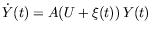(93)

where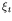is a multiplicative noise wich can model for instance longitudinal turbulence. Using the method described in , the non-Gaussian processis constructed using a truncated Hermite chaos polynomial expansion: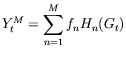(94)

where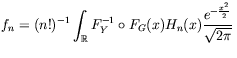d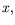(95)

The Lyapounov exponent is finally constructed, using a simulated sample path of equation (93) solution: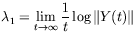(96)

In this paper our goal is to consider the effect of the distribution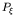on the overall stability. We will consider here two families of distribution, the centered exponential distribution,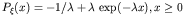and the centered Rayleigh distribution,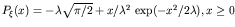. The spectrum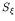will be modeled by the von Karman model. Numerical results show , as it was noticed in [2,4] for Gaussian noise, that if multiplicative noise has an impact on the stability of the linear airfoil, so does the distribution of the multiplicative noise, the more nonGaussian the distribution is the more important the effect. In presence of freeplay, the role of the noise distribution is crucial since, for this particular application, a Gaussian noise does not change significally the stability behaviour of the airfoil.

References
1
B. Puig, F.Poirion and C. Soize, "Non-Gaussian simulation using Hermite polynomial expansion: convergences and algorithms", Prob. Eng. Mech., Vol. 17, No. 3, 253-264, 2002. doi:10.1016/S0266-8920(02)00010-3
2
I. Zentner and F. Poirion, "Stability of fluid-structure coupled systems in the presence of multiplicative and additive noise. Application to airfoil flutter", 7th ICCST proceedings , CDrom, 2004.
3
L. Arnold, Random dynamical systems, Springer Verlag, Berlin Heidelberg 1998.
4
D. Poirel and S. Price, "Response probability structure of a structurally non-linear airfoil in turbulent flow", Probabilistic Engineering Mechanics, Vol. 18, 2003.
5
F. Poirion, "On some stochastic methods applied to aeroservoelasticity", Aerospace Science and Technology, No. 4, 2000, 201-214. doi:10.1016/S1270-9638(00)00118-8

purchase the full-text of this paper (price £20)